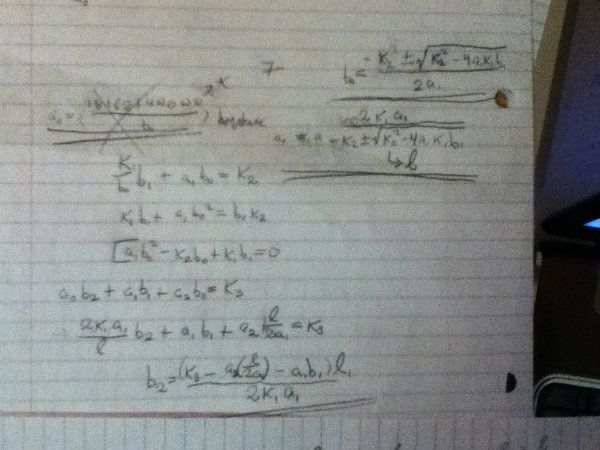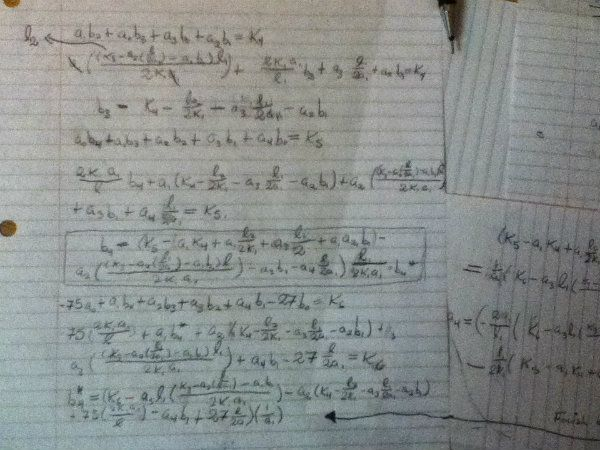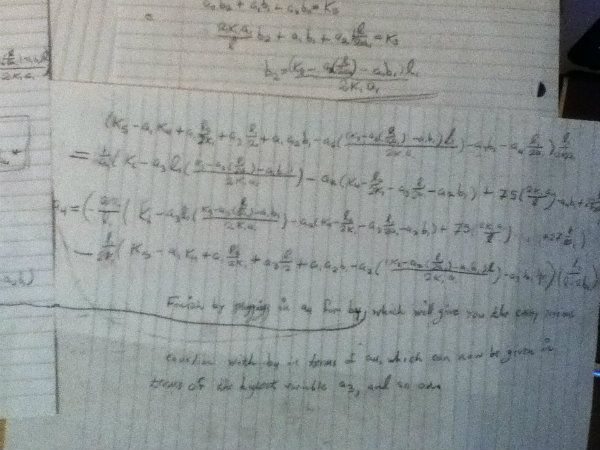User Name Remember Me? Password

 Abstract Algebra Abstract Algebra Math Forum

 May 30th, 2012, 05:49 AM #1 Newbie   Joined: May 2012 Posts: 2 Thanks: 0 Solvability of equation Hi, my computer science problem ends solving of equations and I need to know if there exists way how to solve it. I know it has integer solution. The following system of equations is easy but I need to solve it for situations when the structure of problem is the same but parameters are from a0 to a20 and from b0 to b20. The only way I found is the substitution method, but it fails already for systems with a0 to a4 ... because it goes to very long polynomial equations. a0*b0 = 31680 a0*b1+a1*b0 = -43008 a0*b2+a1*b1+a2*b0 = 22084 a0+a1*b2+a2*b1+b0=-5460 a1+a2*b2+b1 = 685 a2+b2 = -42 For this a bit more complex system I'm not able to find the solution: a0*b0 = 18162144000 a0*b1 + a1*b0 = -37815724800 a0*b2 + a1*b1 + a2*b0 = 34351382160 a1*b2 + a0*b3 + a3*b0 + a2*b1 = -18067743072 a0*b4 + a1*b3 + a2*b2 + a3*b1 + a4*b0 = 6147827896 -75*a0 + a1*b4 + a2*b3 + a3*b2 + a4*b1 - 27*b0 = -1429787040 a0 - 75*a1 + a2*b4 + a3 b3 + a4 b2 - 27 b1 + b0 = 233659985 a1 - 75*a2 + a3*b4 + a4*b3 - 27*b2 + b1 = -27102726 a2 - 75*a3 + a4*b4 - 27*b3 + b2 = 2219703 -75*a4 + a3 + b3 - 27*b4 = -125460 a4 + 2025 + b4 = 4655 Thanks! CyrrusMay 31st, 2012, 06:01 PM #2 Member   Joined: Jul 2010 Posts: 42 Thanks: 0 Re: Solvability of equation There's a theorem that states that if a polynomial is irreducible in where is a prime number, then the polynomial is irreducible in the rational numbers. However, cannot divide the leading coefficient. In your case that's your greatest for . Consider something like and go from there. This helps to determine if whether or not the system of equations actually has a solution in the rational numbers. I'll see if I can help you any further, since you seem sure that it does have a solution. Edit: I was able to use substitution to get to a point where solving it just involved a lot of computation. Substitution should be doable if you use a lot of short-hands and formulas. Here's are some pictures.June 4th, 2012, 12:21 AM #3 Newbie   Joined: May 2012 Posts: 2 Thanks: 0 Re: Solvability of equation Hi Xhin, thanks for your reply. As far I understand the problem I know it goes to 2 situations. The system has solution in R or it doesn't have. I need to distinguish between these two cases. If I find the solution the better but it's not neccessary. I don't understand how to determine the solvability in Z2. Can you send me the scans with some description in better quality to email? I'm not able to read it and follow your idea. cyrrussmith I'm at gmail.com. Thanks again.Tags equation, solvabilityThread ToolsShow Printable VersionEmail this Page Display ModesLinear ModeSwitch to Hybrid ModeSwitch to Threaded ModeSimilar Threads Thread Thread Starter Forum Replies Last Post PhizKid Differential Equations 0 February 24th, 2013 10:30 AM mrkdsmith Linear Algebra 0 October 29th, 2011 01:07 AM raiseit Math Events 1 June 1st, 2010 01:38 AM BSActress Number Theory 8 October 17th, 2009 04:56 PM JC Number Theory 3 August 4th, 2008 07:19 PM

 Contact - Home - Forums - Cryptocurrency Forum - Top